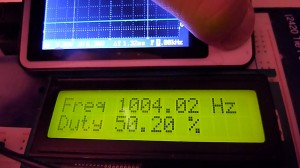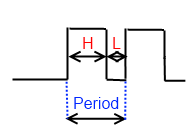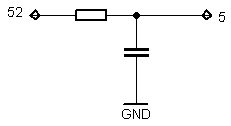# Arduino frequency counter/duty cycle meterThis meter gives the best results at 0 – 1000 Hz  range. It works by measuring square wave total and high period duration using 16 bit hardware counter.As you may know frequency = 1/Period and  Duty Cycle = High period duration/total period duration.

# Very simple Arduino capacitance meter using 16 bit timer and analog comparator

As You could see from video it requires only one external resistor. Meter is very simple, but obviously not very accurate or wide range. Theoretically it could measure in 10 nF – 160 µF range.

How it Works?

Circuit formed from resistor and capacitor (RC circuit) has time constant, it shows time needed to discharge capacitor via resistor to ~37% it’s initial voltage. Calculation of time constant is very simple t=R*C . For 1 k resistor and 47µF capacitor it’s 47ms.My capacitance meter fully charges capacitor, when 16 bit timer is started and capacitor is discharging via resistor (1 k). Capacitor is connected to analog voltage comparator(pin 5), as capacitor voltage drops bellow 1.1 V occurs analog comparator interrupt which triggers timer interrupt.  If resistor is constant, discharge time and capacitance dependency is linear, therefore if You know one rated capacity capacitor discharge time, You could easily calculate another capacitor’s capacitance by measuring time.

Because timer uses only one  /64 clock prescaler measurement range is very narrow. To have wider range there is a always way to make  programmable prescaler, which changes if capacitor value is out of range, or discharge capacitor via range of different resistors.

Also keep in mind that resistor’s resistance depends on environment temperature, consequently and on LCD display showed capacitance. To fix this temperature dependency referenced capacitor should be used. In this case every time both capacitors are charged and discharged separately, but via the same resistor. Capacitance proportion is calculated and if referenced capacitor capacitance is know measured capacitor’s value can be calculated just by division or multiplying.

It’s time for program’s code.